# Precalculus : Functions

## Example Questions

1 2 11 12 13 14 15 16 17 19 Next →

### Example Question #181 : Functions

Find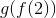given the following functions: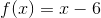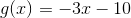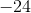Explanation:

To solve, simply plug in 2 into f and then the result into g.

Thus,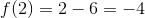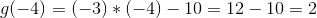### Example Question #1311 : Pre Calculus

Suppose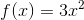and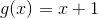.  Find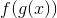.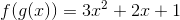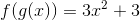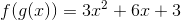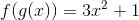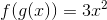Explanation:

To find, you must subsititue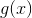into the function,.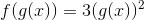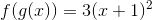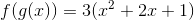### Example Question #181 : Functions

If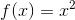and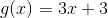, what is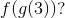Explanation:

First, we find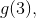or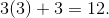Then, find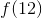, or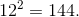### Example Question #1312 : Pre Calculus

Given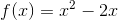and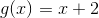find.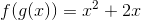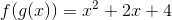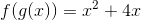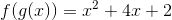None of these.Explanation:

Findingis the same as plugging inintomuch like one would find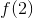for a function.andInsert g(x) into f(x) everywhere there is a variable in f(x):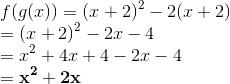### Example Question #185 : Functions

We are given the following: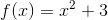and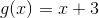.

Find: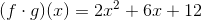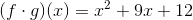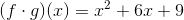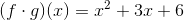Explanation:

Let's discuss what the problem is asking us to solve. The expression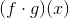(read as as "f of g of x") is the same as. In other words, we need to substituteintoSubstitute the equation offor the variable in the givenfunction:Next we need to FOIL the squared term and simplify: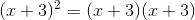FOIL means that we multiply terms in the following order: first, outer, inner, and last.

First: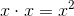Outer: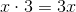Inner: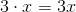Last: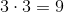When we combine like terms, we get the following: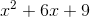Substitute this back into the equation and continue to simplify.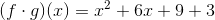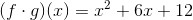None of the answers are correct.

1 2 11 12 13 14 15 16 17 19 Next →

### All Precalculus Resources